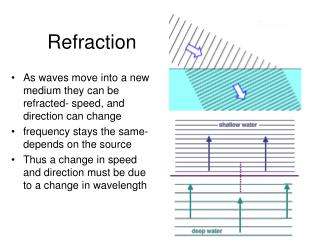DownloadDownload PresentationRefraction

# Refraction

Download Presentation## Refraction

- - - - - - - - - - - - - - - - - - - - - - - - - - - E N D - - - - - - - - - - - - - - - - - - - - - - - - - - -
##### Presentation Transcript

1. Refraction • As waves move into a new medium they can be refracted- speed, and direction can change • frequency stays the same- depends on the source • Thus a change in speed and direction must be due to a change in wavelength

2. Refraction – Soldier Analogy Imagine a line of soldiers marching from a road onto sand They will move more slowly on the sand and the line will bend

3. Direction of Bending • FST- fast to slow: towards the normal • SFA- slow to fast: away from normal • Draw the estimated refracted ray

4. Refraction What Are The Different Media? Water Glass Air

5. Dispersion of light through refraction • Different wavelengths of light refract by different amounts • Thus the prism

6. Mirages • Hot air near surface of road causes bending • Your brain interprets this the only way it knows how- there must be water on the road that is reflecting

7. Quantifying Refraction • n= index of refraction (no units) • n=c/v • c= speed of light in a vacuum • v=velocity of light in the medium • Remember v=f ? • n= f/ 2f • Since f does not change n=/2

8. Light at interface between 2 mediums • When light reaches interface, it generally splits into 2 parts: • Part is reflected (follows law of reflection) • Part is refracted • Refracted ray enters new medium and can change speed, wavelength, and direction

9. Snell’s Law • n1sin1=n2sin2 • All incident and reflected are labeled (1) and all refracted are labeled (2) • Refraction is reversible- you could turn light ray around and it would follow the same path

10. Snell’s Law • In lab, we’ll often use a semicircular tray • Notice how if you aim the incident ray at the center of the flat side, it will exit the tray at the normal to the curved surface • Since  = 0 there will be no refraction at that interface

11. Problem Solving: Snell’s Law • nair=1 • nwater=1.33 • Find the angle of refraction • Check your answer using the FST, SFA rule

12. Snell’s Law: Multiple Interfaces • Snell’s law can be used to go through successive interfaces • Find the angle of refraction within the glass • Find the angle of refraction when it re-enters air nglass=1.52

13. Snell’s Law: prisms • Where does the light exit the glass and at what angle? • Treat it like the previous layer problem but now the layers are not parallel

14. Total Internal Reflection (TIR) • When n1>n2 the refracted ray will bend away from the normal • As you increase 1 you will reach a point where the r=90 • The refracted ray now doesn’t leave the first medium • If you set r=90 and solve for 1 you find the critical angle (c) where TIR occurs

15. TIR • At any angle greater than the critical angle, you will have TIR • Remember it only happens from a higher n into a lower n medium

16. TIR problems • Calculate the critical angle for an ethanol-air boundary. • Draw a diagram of the path of the light ray at the critical angle • nethanol = 1.36 47.3 light travels from ethanol to air

17. Total Internal Reflection Diamonds

18. Total Internal Reflection Fiber Optic Data Cables

19. Total Internal Reflection Rainbow

20. Lenses • Ray tracing for lenses similar to spherical mirrors except light passes through instead of reflecting • Lenses have real or virtual image

21. Converging Lenses • Converging: thicker in middle • Light refracted through real focus • forms REAL, inverted IMAGE

22. Image Formation: Converging Lens • RULES- use the 2 that fit the situation • Incident ray entering parallel is refracted through focus • OR Incident ray entering via the focus is refracted parallel • Ray through center of lens doesn’t bend

23. Diverging Lenses • Diverging: thinner in the middle • Light bends AWAY from a virtual focus on the incident side of the lens • Virtual, upright image

24. Image Formation: Diverging • RULES- use the 2 that fit the situation • Incident ray entering parallel refracted away from virtual focus • Incident ray entering through virtual focus refracted parallel • Incident ray passing through center of lens doesn’t bend

25. Mathematics of Lenses • Similar to Mirrors- uses same equations • BEWARE OF SIGNS SIGN RULES • Converging: f is + • Diverging: f is negative • Si is + for real images • Si is - for virtual images

26. Lens Equation Problem • An object is placed 7.10cm to the left of a diverging lens whose focal length is f=-5.08cm. • Draw ray diagram. • Find the image distance and determine is image is real or virtual. • Find the magnification.

27. solution • 1/si=(1/-5.08) - (1/7.10) • si=-2.96 • Since si is negative, image is virtual and located to the left of the lens • M=-si/so=-(-2.96)/(7.10)= 0.47

28. Problems with multiple lenses • Treat each lens separately- work through them in order • Use the image from the first lens as the object for the 2nd and continue this process until all lenses used